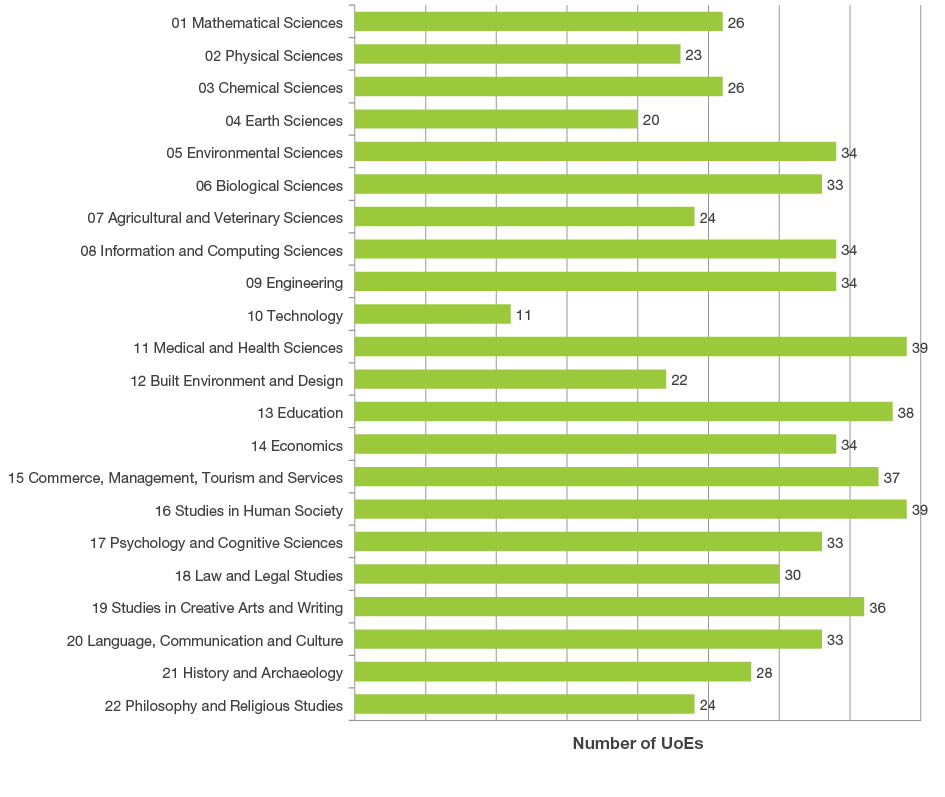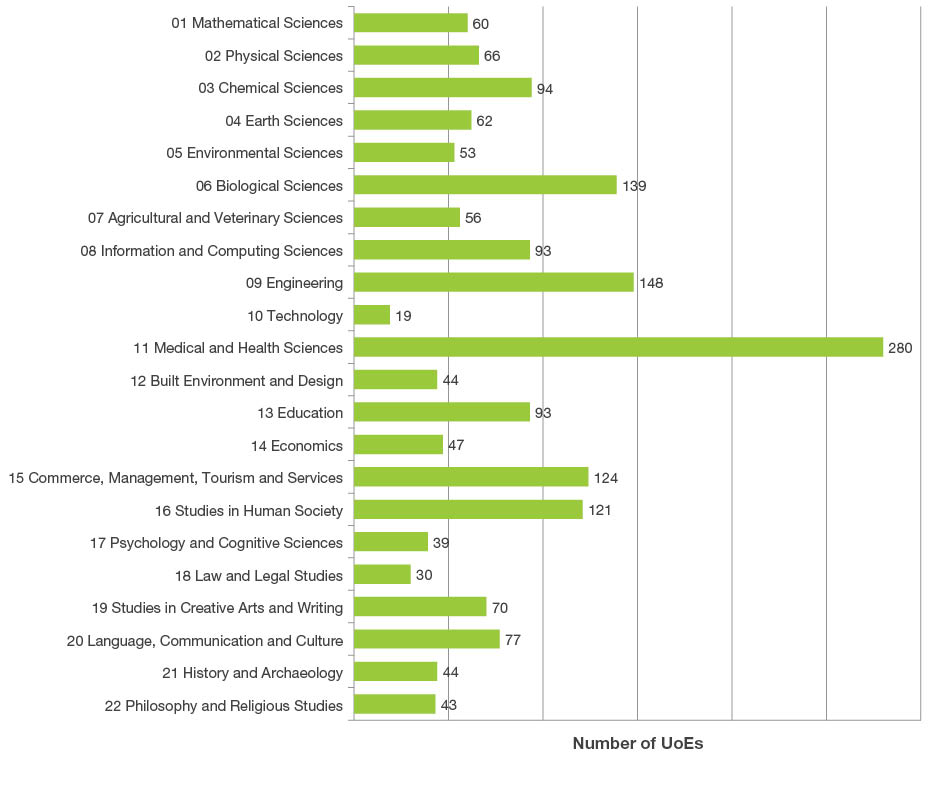Search

# Section 1

## Assessed Units of Evaluation

In ERA, a Unit of Evaluation (UoE) is the research discipline, as defined by the ANZSRC two- and four-digit FoR codes, for an eligible institution (Appendix 1). A UoE is assessed for a specific institution when it has sufficient research volume to be considered research active for the purposes of ERA. ERA evaluation occurs at both the two- and four-digit FoR code levels.

A total of 2,460 (UoEs) were assessed in the ERA 2015 evaluation process. There were 658 two-digit units assessed and 1,802 four-digit units assessed. The first chart below shows the number of two-digit assessed UoEs only. The second chart shows the number of four-digit UoEs assessed aggregated to the two-digit level.

### UoEs assessed by two-digit FoR### UoEs assessed by aggregated four-digit FoR codes (grouped by two-digit FoR code)